# Thue-Mahler equation

(diff) ← Older revision | Latest revision (diff) | Newer revision → (diff)

Letbe a binary form of degree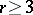, irreducible over, letbe a fixed set of rational prime numbers and let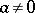be a fixed rational integer. The Diophantine equation (cf. also Diophantine equations)(a1)

in the unknowns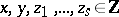, withandrelatively prime, is called a Thue–Mahler equation. More generally, letbe an algebraic number field (cf. Number field; Algebraic number), letbe a fixed finite set of places in(cf. also Place of a field), containing all infinite ones, let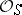be the ring of-integers and let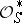be the group of-units of. Let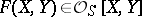be a binary form of degree, irreducible over. The Diophantine equation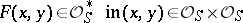(a2)

is called a generalized Thue–Mahler equation. If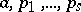are as in (a1) and one takes in (a2)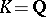and, then all solutions of (a1) are also solutions of (a2). Hence, any result concerning the solutions of (a1) applies also to those of (a2).

In 1933, K. Mahler, using his-adic analogues of the methods of A. Thue [a7] and C.L. Siegel [a5], proved in [a3] that a Thue–Mahler equation (a1) has at most finitely many solutions. Because of the applied methods, this result is non-effective, i.e. it does not imply an explicit bound for either the size of the unknowns, or for the number of solutions. The development of Baker's theory (cf. also Gel'fond–Baker method) and its-adic analogues made possible, in the 1970s, the proof of effective, though not explicit, bounds for the size of the unknowns; see [a4], Chap. 7. Subsequently, very explicit upper bounds for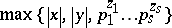have been proved. A characteristic result of this type is due to Y. Bugeaud and K. Gőry [a1], in which the quantities,,,,,,are involved; here,is an upper bound for the absolute values of the coefficients ofand,are, respectively, the class number and the regulator of the number field generated (over) by a root of the polynomial(cf. also Class field theory).

Due to techniques in Diophantine approximations, explicit upper bounds for the number of essentially distinct solutions have been proved for (a2), where two solutions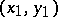,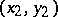are considered as essentially distinct ifis not of the form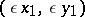for some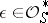. In view of the observation following (a2), such a bound is also valid for the number of solutions of (a1). Thus, Mahler's finiteness result has been considerably generalized and, what is more, in an explicit form. A characteristic result of this type is due to J.-H. Evertse [a2]: Let the cardinality ofin (a2) be. Then, the number of essentially distinct solutions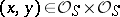is at most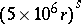.

In the early 1990s, constructive methods for the explicit computation of all solutions of a Thue–Mahler equation (a1) were developed by N. Tzanakis and B.M.M. de Weger [a8], [a9]. These are based on the theory (real and complex as well as-adic) of linear forms in logarithms of algebraic numbers (cf. Linear form in logarithms) and reduction techniques, like the LLL-basis reduction algorithm and the computation of "small" vectors in a lattice (cf. also LLL basis reduction method). This method can, in principle, be extended to equations of the form (a2), as shown by N.P. Smart in [a6].

How to Cite This Entry:
Thue-Mahler equation. Encyclopedia of Mathematics. URL: http://encyclopediaofmath.org/index.php?title=Thue-Mahler_equation&oldid=18261
This article was adapted from an original article by N. Tzanakis (originator), which appeared in Encyclopedia of Mathematics - ISBN 1402006098. See original article